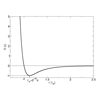#### You may also like### Integration Matcher

Can you match the charts of these functions to the charts of their integrals?### Brimful

Can you find the volumes of the mathematical vessels?### Lennard Jones Potential

Investigate why the Lennard-Jones potential gives a good approximate explanation for the behaviour of atoms at close ranges

# Interpolating Polynomials

##### Age 16 to 18 Challenge Level:

This problem is an extension of secondary ideas, intended primarily for the keen and those considering mathematics at university.

There are many applications of the idea of interpolating polynomials, but this problem is more about presenting the ideas of existence and uniqueness proofs, as well as giving students an intuition for the different ways graphs can be manipulated.

### Discussion ideas

You can also discuss how a polynomial of degree $n$ can be defined in two different ways – either as the $n+1$ coefficients of powers of $x$, or the values of the polynomial at $n+1$ distinct inputs. In linear algebra terminology, such polynomials belong to an $n+1$-dimensional vector space. A more intuitive notion of dimension that may be more suitable at this level is a measure of "free"-ness: add a dimension for each (real-valued) free variable and subtract one for each constraint.